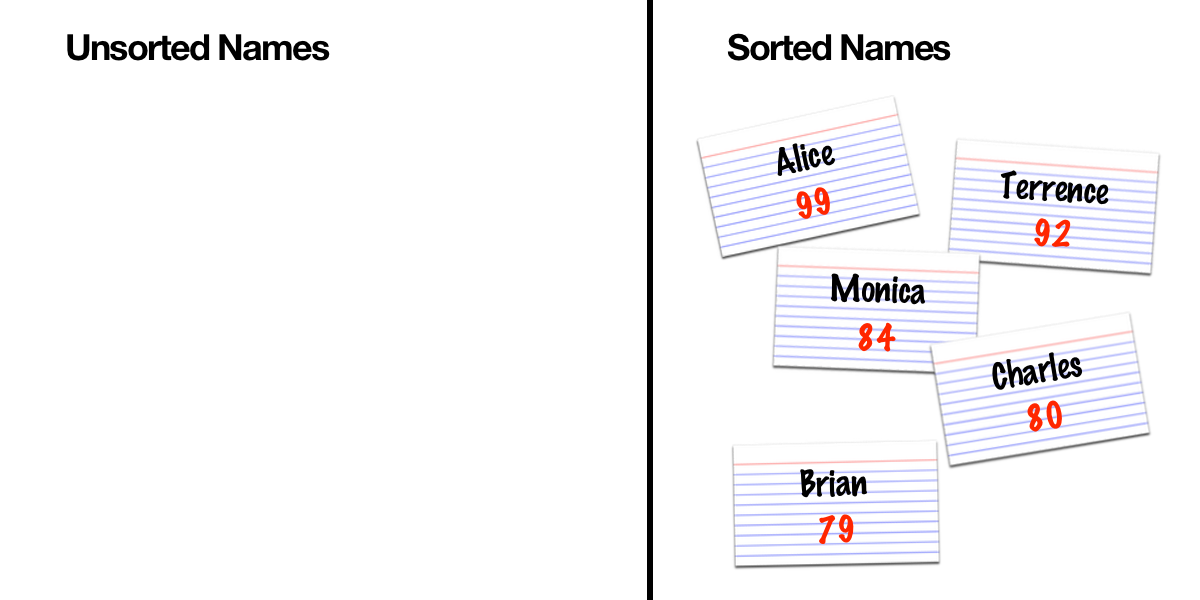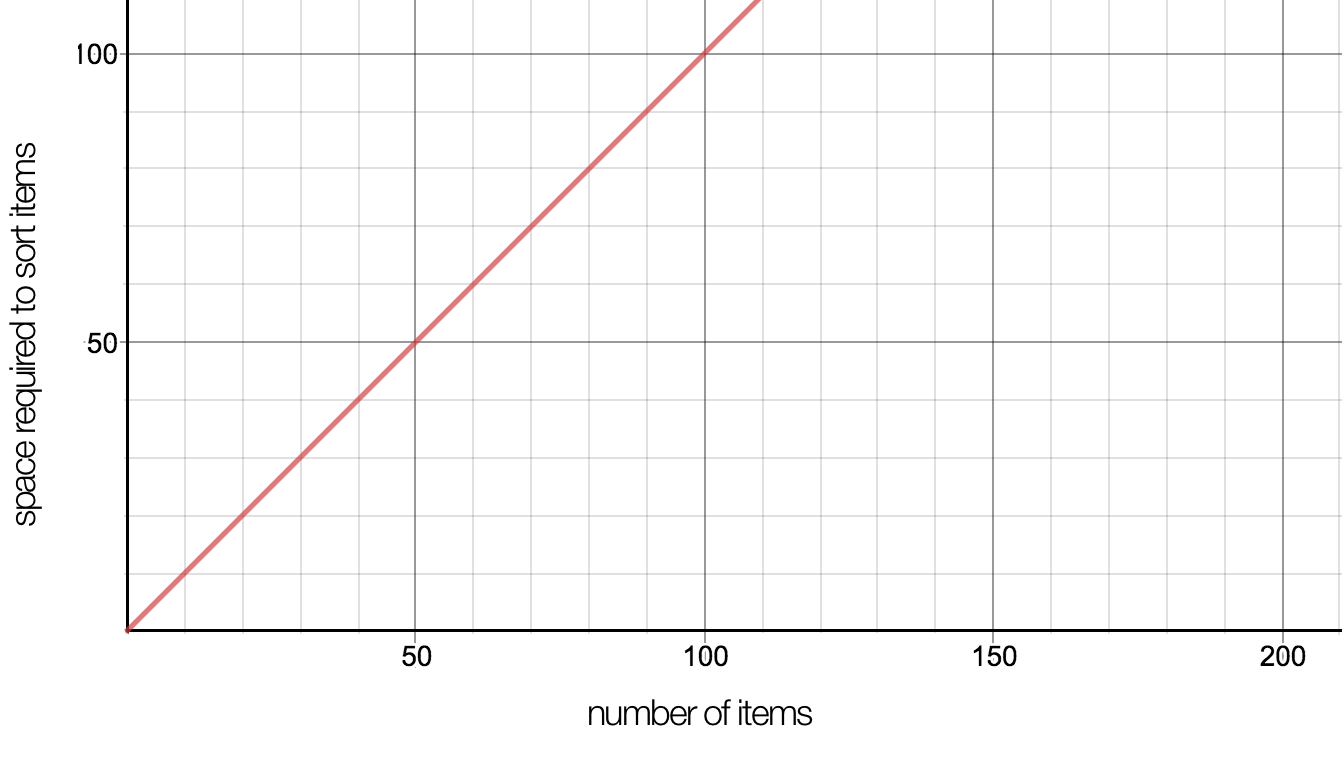# Sorting Things Out (Part 1)

Sorting is the process of arranging or ordering things based on a specific property. For example, numbers can be ordered by their value: 1 is smaller than 2. If we order the list by ascending order, 1 comes before 2. We can also sort a list of numbers by descending order. In that case, 2 comes before 1.

However, sorting also occurs in the physical world. For example, we can sort objects by size. In the United States, Coinstar machines are vending machines that automatically sort and count coins for you. They charge a fee for this service, but let’s see how it actually works.

The key to sorting coins is that each coin is a different size. Inside a coin-sorting machine, there are different sized holes: one for each coin. If you’re curious how this works, go ahead and watch this video:

The process of sorting the coins makes it much easier to count change. Similarly, in computer science, the process of sorting items makes it much easier to apply other algorithms, such as searching and merging large amounts of data.

# Why bother learning how to sort in the first place?

In practice, every popular programming language has a built-in sort function:

In my binary search article, we saw how the binary search algorithm can be used to find an item extremely quickly. However, it only works if the input is sorted. Binary search relies on the property of ordering to discard half of the input at every step. Without sorted input, the desired item could be in either half!

Another motivation to learn how to sort is because sorting is a problem with many well-documented implementations. There’s the bubble sort, the insertion sort, the selection sort, the merge sort, the quicksort, and many other variations. Some of those implementations are more efficient, so by studying the solutions that already exist, we can learn the techniques that make certain algorithms faster than others.

In this article, we’ll take a look at an approach called selection sort because it mirrors the way humans sort objects in real life.

# How do we sort things in real life?

Let’s imagine that we’re judging a competition. We have a pile of name cards with each person’s current score and now we want to find out who the top competitors are. To do this, we need to sort the competitors by their score.

We should probably start by reserving some space to put the sorted names. Now we have two piles: the unsorted pile and the sorted pile (which is currently empty).

One way to do this is by looking at all of the names in the unsorted pile and finding the one with the highest score. Once we’ve found the highest score, we move it to the sorted pile.

In this case, Alice has the highest score, so we’ll move her card over to the sorted pile.

Now we go through the unsorted pile, find the person with the highest score, and move them over the sorted pile.

The next step is to go back through the unsorted pile, find the person with the highest score, and move them over to the sorted pile. This is starting to sound repetitive, isn’t it? When we sort cards in real life, we repeat this step until there are no more cards in the unsorted pile.Repeat the process of picking the person with the highest score and moving their card over to the sorted pile

Now that all the cards are sorted, let’s take a look at the performance of this approach.

# Performance

There are two primary aspects of performance that computer scientists are usually interested in: space and time.

• Space complexity is how much memory is used when the program runs.
• Time complexity is how long it takes to run the program.

## Space Complexity

The first thing to notice when we sorted the cards is that we used two piles for the unsorted names and the sorted names. In the physical world, this would mean that we need twice the amount of desk space to sort the cards. If we translate this process directly into a computer program, we would need to use two arrays, or 2x the amount of memory.

If we want to be conservative with space, we could make optimizations to this process by sorting the cards in-place. Starting with the unsorted pile, we do the same thing as before, but this time, we find the highest score and shuffle the cards around so that we don’t need extra desk space.

Let’s start with the first card and replace it with the highest score. Instead of clearing off extra desk space, we partition the original pile into a sorted part and an unsorted part (using the same amount of space as the original pile).

Now that we know the first card has the highest score, we move onto the second card and swap it with the highest score from the unsorted part.

We’ll repeat this process until we’ve finished swapping every card in the original pile with the highest score. Each time that we swap a card, the sorted part grows and the unsorted part shrinks.

We’ll end up with something that looks like this:

If there are n cards in the original pile, then we would say that this implementation has O(n) space complexity.

If you’re new to Big-O Notation, Justin Abrahms wrote a helpful post called “Big-O notation explained by a self-taught programmer”. If O(n) is confusing, feel free to take a break and read Justin’s article.

O(n) space complexity means that if we’re sorting n items, we also need the same amount of space to sort those items.The amount of space required increases linearly with the number of items

To help us visualize this, let’s apply the constraint that the cards are not allowed to overlap. That means the amount of desk space that we need is the amount of space that’s required to place 5 cards without overlapping.

If we translate this idea to a computer program, we might use an array with 5 slots: one for each card. That’s where O(n) comes from. For n cards, we need n spaces to perform the sort.

## Time Complexity

The key to our algorithm is that for each card in the unsorted pile, find the person with the highest score, and move them over to the sorted pile.

Let’s unpack this statement.

“For each card in the unsorted pile” means that we need to perform some action for every item in the input. If there are n items in the pile, we have to perform n actions. In Big-O notation, we would say that this takes O(n) time.

Now let’s take a look at the action that needs to be performed for every item.

“Find the person with the highest score” means that we have to look at every card to pick the one with the highest score. If we have 5 items in the unsorted pile, we have to look at all 5 of them to determine which one actually has the highest score… but we have to repeat this process for every card in the unsorted pile!

This means that we actually have to look at every card n*n (or ) times. In Big-O notation, we would write this as O(n²).

However, there’s one more part to analyze.

“Move them over to the sorted pile” is the act of moving or swapping the cards. Within a computer program, moving or swapping can be implemented as variable assignment, which is a constant time operation. A constant time operation is something that always takes the same amount of time.

Even though we eventually have to move every card over to the sorted pile, Big-O notation is concerned with the worst case, which is already O(n²). Constant time operations would not change the worst case runtime, so we just say that our algorithm runs in O(n²) time.

Now that we’ve explored a real world example, let’s implement selection sort!

# Stepping Through The Algorithm

For every item in the unsorted list, we want to find the smallest element in the rest of the list. Once we find it, we’ll swap the current item with the smallest element.

Let’s step through this algorithm with an example.

• The first item is 99, and the rest of the items are [79, 84, 80, 92]
• The smallest item in the rest of the list is 79
• Now we swap the current item (99) with the smallest item (79)
• The list now looks like this: [79, 99, 84, 80, 92]
• At this point, the first item in the list is the smallest item in the list.

Now we repeat this for every other item in the list:

• The second item in the list is 99, and the rest of the items are [84, 80, 92]
• The smallest item in the rest of the list is 80
• Now we swap the current item (99) with the smallest item (80)
• The list now looks like this: [79, 80, 84, 99, 92]

Notice that as we iterate through the list, we begin to notice that the part we’ve iterated through is sorted, so we can visualize the list as having a sorted part and an unsorted part:

• Sorted part: [79, 80]
• Unsorted part: [84, 99, 92]

This is a lot like how we were shuffling the cards in place on the table!

Before we look at code, let’s finish tracing the algorithm:

• The next item in the list is 84, and the rest of the items are [99, 92]
• The smallest item in the rest of the list is still 84
• Even if we swap the current item (84) with the smallest item (84), the list looks the same: [79, 80, 84, 99, 92]

At this point the sorted part is [79, 80, 84] and the unsorted part is [99, 92].

• The next item in the list is 99, and the rest of the items are 92
• The smallest item in the rest of the list is 92
• Now we swap the current item (99) with the smallest item (92)
• The list is now [79, 80, 84, 92, 99]

We just stepped through the whole algorithm, so let’s see what this might look like in code.

# Implementation

The following implementation is written in Python because the language has a lot of built-in features that make it easy to read.

In Part 2, we’ll explore the limitations of the selection sort algorithm and discover some new ways to improve on what we’ve learned.

This is part of a series that I’m creating to teach the fundamentals of computer science and algorithms in a way that’s approachable to people without a computer science background.

--

--